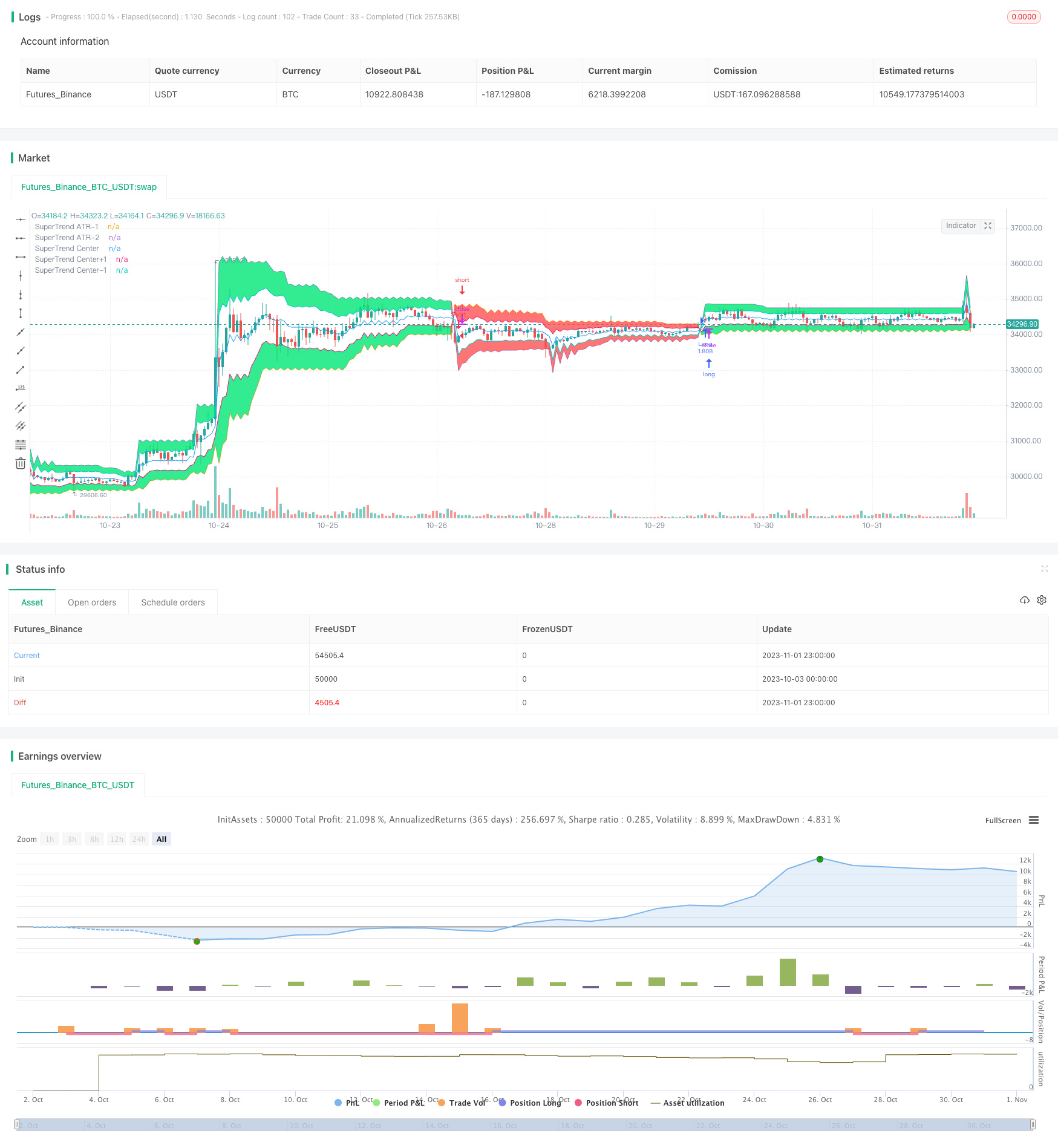# Beyond The Clouds Strategy

Author: ChaoZhang, Date: 2023-11-03 16:10:33
Tags:## Overview

This strategy uses the Super Trend indicator to assist in placing orders, and filters by cloud layers and candlestick colors to place limit orders for increasing profitability. Its goal is to quickly capture trends after they start, and reduce risk during consolidation.

## Strategy Logic

1. Calculate the average of highest and lowest prices within ATR period as the baseline.

2. Calculate the upper and lower bands based on the Factor multiplier.

3. When close is above upper band, mark as 1; below lower band, mark as -1. Otherwise, maintain previous state.

4. Dynamically adjust stop loss line based on close price’s position relative to upper/lower bands.

5. Calculate cloud layer range based on a certain percentage of upper/lower band interval.

6. For long, need close < open when Super Trend is 1. For short, need close > open when Super Trend is -1.

7. Place limit buy orders at previous bar’s close price for long. Place limit sell orders for short.

8. Filter by time range, close all positions available.

This strategy combines Super Trend and cloud concept, which allows fast trend capturing after trend starts. Super Trend stop loss responds faster than normal moving stop loss. Cloud layers avoid losses from false breakouts. Limit orders reduce slippage and increase profitability. The main advantages are:

1. Super Trend is sensitive and tracks trends strongly.

2. Cloud layers filter reduces losses from false breakouts.

3. Candlestick color helps avoid reversals.

4. Limit orders decrease slippage impact and increase win rate.

5. Customizable time range and position management suit different trading needs.

## Risk Analysis

There are also some risks to note:

1. Improper Super Trend parameters may cause too much sensitivity and whipsaws.

2. Excessive cloud range may filter out valid breakout signals, impacting profitability.

3. Limit orders may not get filled during high volatility, missing opportunities.

4. No stop loss can fully avoid systemic risk and huge losses.

5. Larger position sizes also amplify losses. Need to control risks.

## Optimization Directions

This strategy can be improved in the following aspects:

1. Test different markets and instruments for optimal Super Trend parameters.

2. Dynamically adjust stop loss level based on market volatility.

3. Optimize cloud range to balance noise filtering and signal retention.

4. Add position sizing module to dynamically size positions based on market conditions.

5. Use different parameter sets for different trading sessions to adapt to market rhythms.

6. Test effectiveness when combining with other indicators.

## Conclusion

In conclusion, this strategy has clear logic and obvious advantage in trend catching. But no strategy can completely avoid systemic risks. Need to control position sizing, keep optimizing to minimize risks in live trading, and maximize the edge. This strategy has great potential for further testing and enhancements to adapt to evolving market dynamics.

```/*backtest
start: 2023-10-03 00:00:00
end: 2023-11-02 00:00:00
period: 1h
basePeriod: 15m
exchanges: [{"eid":"Futures_Binance","currency":"BTC_USDT"}]
*/

//Noro
//2018

//@version=2
strategy("Noro's SuperTrend Strategy v2.0 Limit", shorttitle = "STL str", overlay = true, default_qty_type = strategy.percent_of_equity, default_qty_value = 100, pyramiding = 0)

//Settings
needlong = input(true, defval = true, title = "Long")
needshort = input(true, defval = true, title = "Short")
capital = input(100, defval = 100, minval = 1, maxval = 10000, title = "Lot, %")
cloud = input(25, defval = 25, minval = 5, maxval = 50, title = "cloud, % of ATR")
Factor = input(title = "Super Trend", defval = 3, minval = 1, maxval = 100)
ATR = input(title = "ATR", defval = 7, minval = 1,maxval = 100)
centr = input(true, defval = true, title = "need center of ATR?")
border = input(false, defval = false, title = "need border?")
fromyear = input(1900, defval = 1900, minval = 1900, maxval = 2100, title = "From Year")
toyear = input(2100, defval = 2100, minval = 1900, maxval = 2100, title = "To Year")
frommonth = input(01, defval = 01, minval = 01, maxval = 12, title = "From Month")
tomonth = input(12, defval = 12, minval = 01, maxval = 12, title = "To Month")
fromday = input(01, defval = 01, minval = 01, maxval = 31, title = "From day")
today = input(31, defval = 31, minval = 01, maxval = 31, title = "To day")

//Super Trend ATR 1
src = close
Up=hl2-(Factor*atr(ATR))
Dn=hl2+(Factor*atr(ATR))
TUp=close>TUp? max(Up,TUp) : Up
TDown=close<TDown? min(Dn,TDown) : Dn
Trend = close > TDown ? 1: close< TUp? -1: nz(Trend,1)
Tsl1 = Trend==1? TUp: TDown
Tsl2 = Trend==1? TDown: TUp
limit = (Tsl1 - Tsl2) / 100 * cloud
upcloud = Tsl1 - limit
dncloud = Tsl2 + limit

//Cloud
linecolor = Trend == 1 ? green : red
centercolor = centr == true ? blue : na
cloudcolor = Trend == 1 ? green : red
cline = (Tsl1 + Tsl2) / 2
P1 = plot(Tsl1, color = border == false ? na : linecolor , style = line , linewidth = 1,title = "SuperTrend ATR-1")
P2 = plot(Tsl2, color = border == false ? na : linecolor , style = line , linewidth = 1,title = "SuperTrend ATR-2")
P3 = plot(cline, color = centercolor , style = line , linewidth = 1,title = "SuperTrend Center")
P4 = plot(upcloud, color = border == false ? na : linecolor , style = line , linewidth = 1,title = "SuperTrend Center+1")
P5 = plot(dncloud, color = border == false ? na : linecolor , style = line , linewidth = 1,title = "SuperTrend Center-1")
fill(P1, P4, color = linecolor == red ? red : lime, transp = 50)
fill(P2, P5, color = linecolor == red ? red : lime, transp = 50)

//Signals
up = 0.0
dn = 0.0
up := Trend != 1 ? 0 : Trend == 1 and close < open ? close : up
dn := Trend != -1 ? close * 1000 : Trend == -1 and close > open ? close : dn